# Collapse of a rectangular visco-plastic heap over a slope

## Problem

As a test case we propose the Balmforth collapse of a heap of a 1D non-Newtonian Bingham fluid over a slope S=-Z'_b (S>0). We see the front moving to the right, and the left front going slowly up hill to the left. The flow is of total thickness h. The Y represents the hight at which the stress is equal to the yield stress, hence there is a shear flow of thickness Y; covered by a plug flow of thickness h-Y. Finaly, the flow should stop.

## Equations

Using lubrication theory Liu & Mei obtained the flux Q and used conservation of mass, this has been reformulated by Balmforth.

Explicit resolution of \displaystyle \frac{\partial h}{\partial t}+ \frac{\partial Q(h)}{\partial x}=0, \; \text{with} \;\; Q = \frac{Y^2}{6} (3 h - Y) (-Z'_b - \frac{\partial h}{\partial x}) \;\text{and} \;\; Y= \text{max}( h - \frac{B}{|S - \frac{\partial h}{\partial x}|} ,0) this is Balmforth formulation. This formulation is more simple than the Liu & Mei’s one (or Hogg). Note a new formulation by Saramito (to be tested). See Bingham simple example for the derivation.

It can be written for Herschel–Bulkley as well.

The numerical algorithm is very trivial (based on heat equation) and centrered here, it can be inproved (see Fernandez-Nieto et al.).

## Code

mandatory declarations:

#include "grid/cartesian1D.h"
#include "run.h"

definition of the height of interface h, the yield surface Y, its O(\Delta) derivative, ‘hp’ and it O(\Delta^2) derivative hpc, a kind of viscosity \nu, dQ, the divergence of Q, time step, value of the slope, of the Bingham parameter, max time step, increment of time step (for output)

scalar h[];
scalar Y[];
scalar Q[];
scalar hp[],hpc[],nu[];
scalar dQ[];
double dt,S,B,tmax,inct=0.0001;
char s;

Main with definition of parameters

int main() {
L0 = 2.;
X0 = -L0/2;
S = 1;
N = 128;
DT = (L0/N)*(L0/N)/5 ;
tmax = 200;

a loop for the three values of Bingham parameter

 for (B = 0.5 ; B <= 2 ; B += 0.75){ //  B = 1.25;  //.5 1.25 2
sprintf (s, "x-%.2f.txt", B);
FILE * fp = fopen (s, "w");
fclose(fp);
sprintf (s, "shape-%.2f.txt", B);
FILE * fs = fopen (s, "w");
fclose(fs);
inct = DT;
run();
}
}

initial elevation: a “rectangular column” of surface 0.5:

event init (t = 0) {
foreach(){
h[] = (fabs(x)<.25) ;
Q[]=0;
}
boundary ({h,Q});
}

print data

//event printdata (t += 1; t <= 100) {
event output (t += inct; t < tmax) {
double  xf=0,xe=0;
inct = inct*2;
inct = min(1,inct);

tracking the front and the end of the heap

  foreach(){
xf = h[] > 1e-4 ?  max(xf,x) :  xf ;
xe = h[] > 1e-4 ?  min(xe,x) :  xe ;
}

save them

  sprintf (s, "front-%.2f.txt", B);
FILE * f = fopen (s, "w");
foreach()
fprintf (f, "%g %g %g \n", fmin((x-xf),0), h[], xe-xf);
fclose(f);
fprintf (stderr, "%g %g %g \n", t, xf, xe);
sprintf (s, "x-%.2f.txt", B);
FILE * fp = fopen (s, "a");
fprintf (fp, "%g %g  \n", t, xf);
fclose(fp);
}

save the hight the flux and the yield surface as a function of time

event printdata (t = {0, 0.0625, 0.25, 1, 4, 100 }  ){
sprintf (s, "shape-%.2f.txt", B);
FILE * fp = fopen (s, "a");
foreach()
fprintf (fp, "%g %g %g %g %g \n", x, h[], Q[], Y[], t);
fprintf (fp, "\n");
fclose(fp);
}

integration

double FQ(double h,double Y)
{
return 1./6*(3*h - Y)*Y*Y ;
//return (2*(2* pow(h - Y ,2.5) + pow(h,1.5)*(-2*h + 5*Y)))/15.;
//return (2*( h* pow(h,1.5)))/5.;
//
}

event integration (i++) {
double dt = DT;

finding the good next time step

  dt = dtnext (dt);

O(\Delta) down stream derivative

  foreach()
hp[] =  ( h[0,0] - h[-1,0] )/Delta;
boundary ({hp});

Centered derivative for h' used in the yield criteria for the yield surface Y

Maybe not the best choice…

  foreach()
hpc[] =  ( h[1,0] - h[-1,0] )/2/Delta;
boundary ({hp});

Yield surface

  foreach()
Y[] =  max( h[] - B/fabs(S -  hpc[])  ,0);
boundary ({Y});

the flux is taken with the mean value with the next cell, \nu is an intermediate variable, a kind of viscosity (that is why we center)

  foreach()
nu[] =   (FQ(h[-1,0],Y[-1,0]) + FQ(h[0,0],Y[0,0]))/2;
boundary ({nu});
foreach()
Q[] = - nu[] * (-S + hp[]) ;
boundary ({Q});

derivative O(\Delta) up stream like for heat equation

  foreach()
dQ[] =  ( Q[1,0] - Q[0,0] )/Delta;
boundary ({dQ});

update h

  foreach(){
h[] +=  - dt*dQ[];
}
boundary ({h});
}

## Run

Then compile and run:

qcc  -g -O2 -DTRASH=1 -Wall bingham_collapse_noSV.c -o bingham_collapse_noSV
./bingham_collapse_noSV > out

make bingham_collapse_noSV.tst;make bingham_collapse_noSV/plots
make bingham_collapse_noSV.c.html
source ../c2html.sh  bingham_collapse_noSV

## Results

which gives h(x,t) and Y(x,t) plotted as a function of x at t={0, 0.0625, 0.25, 1, 4, 100, 400 }

  set term png ;   set output 'cmp.png';
set ytics font ",8"
set multiplot layout 3,1 title "collapse of Bingham 1D on a slope"
set xlabel "x"
p[-0.4:1.][:1.6]'shape-0.50.txt' t 'B=0.50 h' w l,''u 1:4 t'Y' w l
p[-0.4:1.][:1.6]'shape-1.25.txt' t 'B=1.25 h' w l,''u 1:4 t'Y'w l
p[-0.4:1.][:1.6]'shape-2.00.txt' t 'B=2.00 h' w l,''u 1:4 t'Y'w l

unset multiplot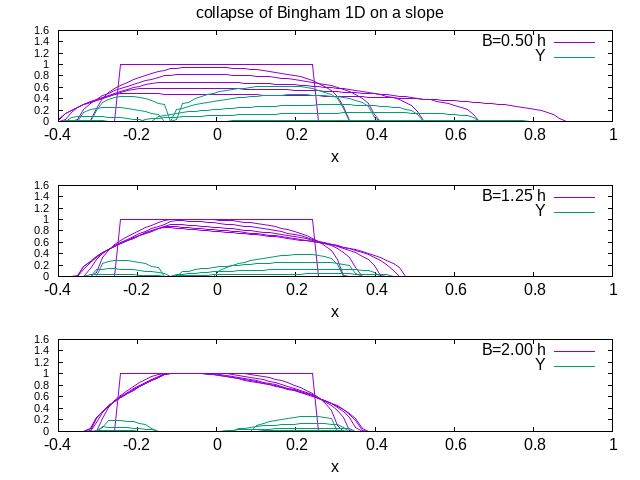(script)

We notice some small differences, the early collapse seems to be more rapid here than in Balmforth.

We now compare with the analytical steady solution of Liu & Mei steady shape. \displaystyle (x -x_{f/e})S = h \pm \frac{B}{S}Log(\frac{B-(\pm S h)}{B}), the front and the end of the final heap compare well

  set term png ;   set output 'std.png';
set font ",8"
set multiplot layout 3,1 title "collapse of Bingham 1D on a slope, final shape compared to Liu & Mei "
set xlabel "x-xf"
set ylabel "h(x,t)  "
B=0.5
p[-1.5:.5][:1]'front-0.50.txt' t 'B=0.50' w l,\
''u (($2) + B*log((B-$2)/B) ):2 t'exact' w l,''u (($2)+$3 - B*log((B+$2)/B) ):2 t'exact' w l B=1.25 p[-1.5:.5][:1]'front-1.25.txt' t 'B=1.25' w l,\ ''u (($2) + B*log((B-$2)/B) ):2 t'exact' w l,''u (($2)+$3 - B*log((B+$2)/B) ):2 t'exact' w l
B=2.0
p[-1.5:.5][:1]'front-2.00.txt' t 'B=2.00' w l,\
''u (($2) + B*log((B-$2)/B) ):2 t'exact' w l,''u (($2)+$3 - B*log((B+$2)/B) ):2 t'exact' w l unset multiplot(script) plot of the front as function of time for the three values of Bingham parameter, the heap is nearly steady at the end…  set term png ; set output 'frt.png'; set xlabel "t" set ylabel "xf " set logscale x p[][:1]'x-0.50.txt' t 'B=0.50' w l,'x-1.25.txt' t 'B=1.25' w l,'x-2.00.txt' t 'B=2.00' w l,.25+sqrt(x)/4 reset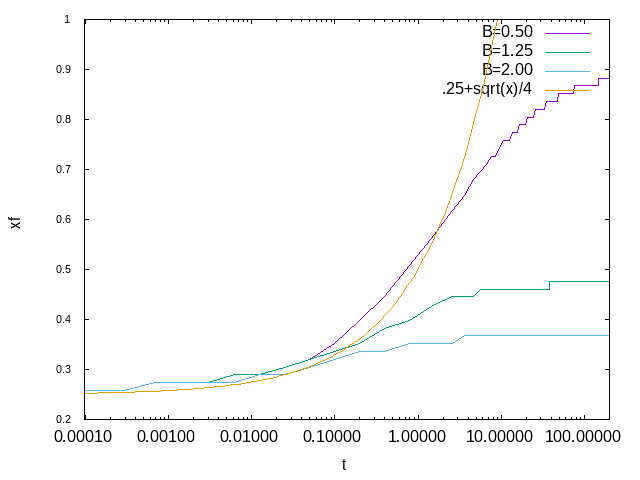(script) ## compare with Balmforth Results Here the figure extracted from Balmforth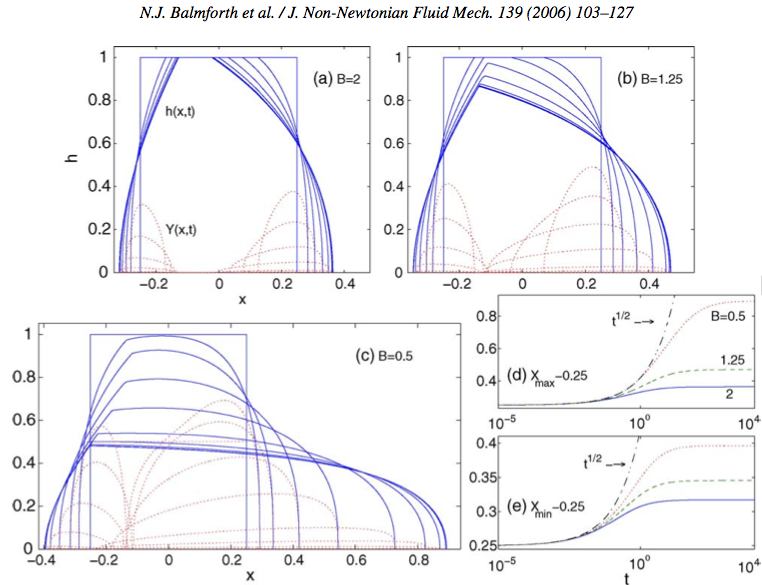the results may be improved if we increase the number of points. note that Y has always some imprecision linked to the inverse in its definition. reset set term png ; set output 'B2.png'; X0=218 X1=369 Y0=313 Y1=526.87 unset tics p[111:370][313:540]'../Img/balmf06.png' binary filetype=png with rgbimage not,\ 'shape-2.00.txt' u (X0+($1/(0.49))*(X1-X0)):(($1>-60)?Y0+$2*(Y1-Y0):NaN) t 'h(x,t)' w lp,\
'shape-2.00.txt' u (X0+($1/(0.49))*(X1-X0)):(($1>-60)?Y0+$4*(Y1-Y0):NaN) t 'Y(x,t)' w lp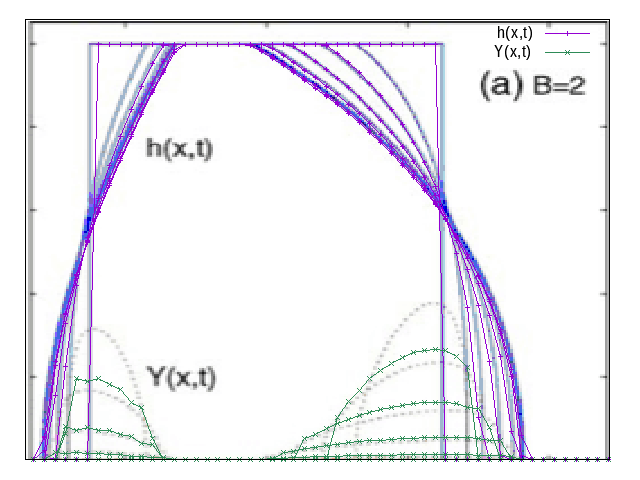h and Y comparison B=2 (script) cas B=0.5 reset set term png ; set output 'Bp5.png'; X0=170 X1=460 Y0=36.53 Y1=249 unset tics p[37:460][34:262]'../Img/balmf06.png' binary filetype=png with rgbimage not,\ 'shape-0.50.txt' u (X0+($1/(0.90))*(X1-X0)):(($1>-60)?Y0+$2*(Y1-Y0):NaN) t 'h(x,t)' w lp,\
'shape-0.50.txt' u (X0+($1/(0.90))*(X1-X0)):(($1>-60)?Y0+$4*(Y1-Y0):NaN) t 'Y(x,t)' w lp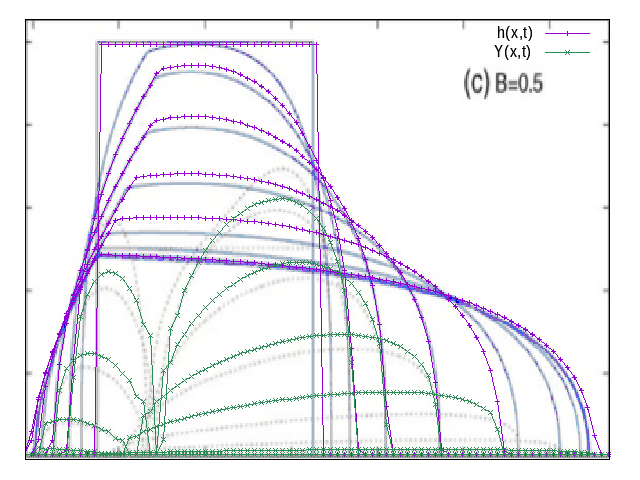h and Y comparison B=.5 (script) cas B=1.25 reset set term png ; set output 'B1p25.png'; X0=522.4 X1=681 Y0=311.6 Y1=526.87 unset tics p[406:694][311:538]'../Img/balmf06.png' binary filetype=png with rgbimage not,\ 'shape-1.25.txt' u (X0+($1/(0.51))*(X1-X0)):(($1>-60)?Y0+$2*(Y1-Y0):NaN) t 'h' w lp,\
'shape-1.25.txt' u (X0+($1/(0.51))*(X1-X0)):(($1>-60)?Y0+$4*(Y1-Y0):NaN) t 'Y' w lp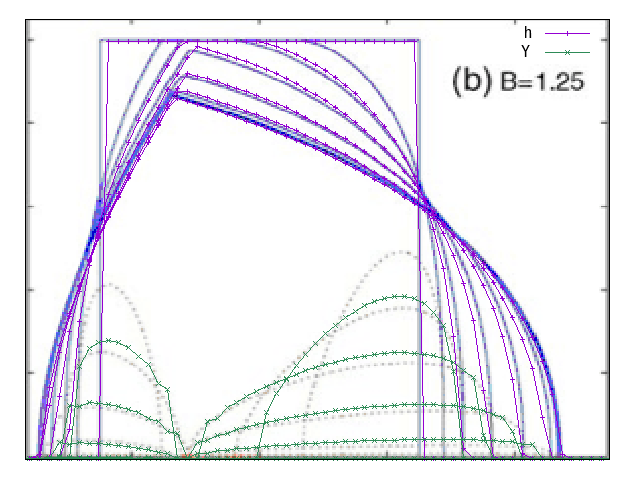h and Y comparison B=1.25 (script) idem  reset set term png ; set output 'B1p25bis.png'; X0=522.4 X1=681 Y0=311.6 Y1=526.87 unset tics p'../Img/balmf06.png' binary filetype=png with rgbimage not,\ 'shape-1.25.txt' u (X0+($1/(0.51))*(X1-X0)):(($1>-60)?Y0+$2*(Y1-Y0):NaN) t 'h' w lp,\
'shape-1.25.txt' u (X0+($1/(0.51))*(X1-X0)):(($1>-60)?Y0+$4*(Y1-Y0):NaN) t 'Y' w lp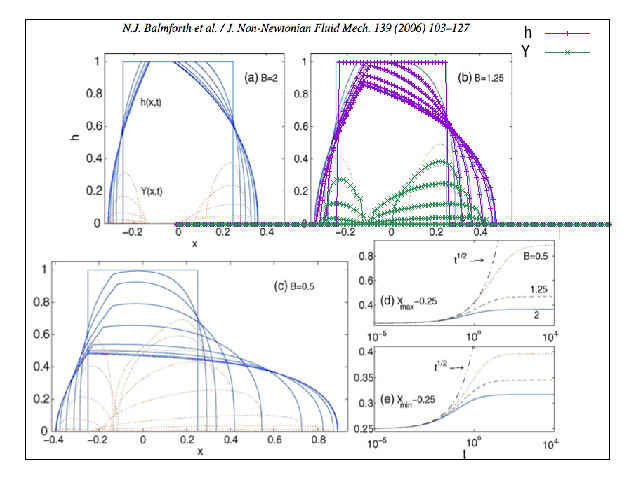h and Y comparison B=1.25 (script) Runout set term png ; set output 'r.png'; X0=655 X1=810 Y0=132 Y1=310 unset tics set key center top plot [475:756][155:290]'../Img/balmf06.png' binary filetype=png with rgbimage not,'x-0.50.txt' u (X0+((log($1))/(10))*(X1-X0)):(((log($1))>-60)?Y0+$2*(Y1-Y0):NaN) w l lw 3 t 'B=0.5','x-1.25.txt' u (X0+((log($1))/(10))*(X1-X0)):(((log($1))>-60)?Y0+$2*(Y1-Y0):NaN) w l lw 3 t 'B=1.25','x-2.00.txt' u (X0+((log($1))/(10))*(X1-X0)):(((log($1))>-60)?Y0+$2*(Y1-Y0):NaN) w l lw 3 t 'B=2'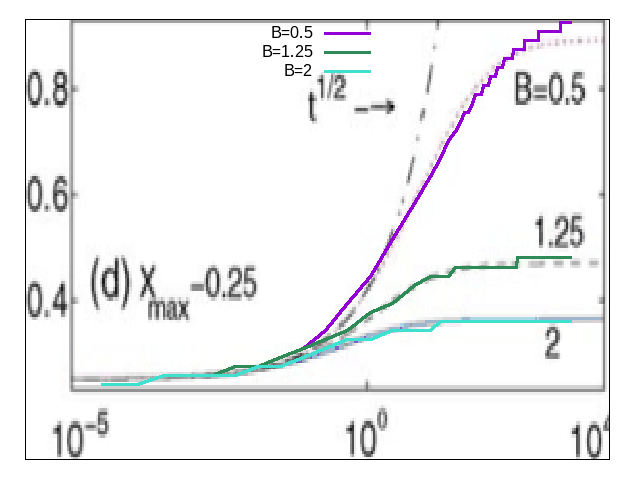runout as function of B (script)